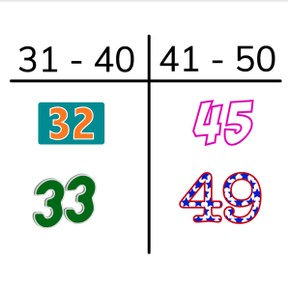Placement of numbers to 50 within tens numbers

# Placement of numbers to 50 within tens numbers

Placement of numbers to 50 on the number line within tens numbers8,000 schools use Gynzy92,000 teachers use Gynzy1,600,000 students use Gynzy

## General

Students learn to place numbers to 50 on the number line within tens numbers.

-1.NBT.B.2

## Relevance

Discuss with students that it is important to be able to place numbers within their tens numbers. You can use this to determine if a number is bigger or smaller.

## Introduction

Show the students a ball. Say that you will tell them a number between 0 and 50 and throw the ball to a student. They must count forwards from the given number and then throw the ball to another student. They continue counting forward and throwing until they reach 50. You can repeat with having them count backwards.

## Development

To place numbers within their tens numbers, students must know what tens numbers are. Explain that a tens is a group of ten. Show the numbers from 1 to 50. Ask the students if they notice anything. Do they notice that all the tens places of the numbers have the same color? When a number has two places, tens and ones, you can see from the number in the tens place what the tens number is. Show the students rows which show the numbers within a ten. Drag numbers to their correct tens boxes. Emphasize that students should look at the tens place of a number to discover the tens. Next circle the numbers that don't sit within the given tens numbers.

Check that students understand placing numbers to 50 on the number line within tens numbers by asking the following questions:
- What are tens numbers?
- What do you look at to determine which tens number a given number is in?

## Guided practice

Students practice placing numbers within their tens numbers. They practice by dragging numbers to boxes showing tens numbers. They then need to drag multiple tens numbers to their correct positions.

## Closing

Discuss again with students that it is important to be able to place a number within its tens numbers because you then know where a number belongs. Check that students understand tens numbers and that they know to look at the tens place of a number to determine where it should go. Prepare sheets of paper with 0-10, 11-20, 21-30, and 31-40) and hang them in the classroom. Hand out number cards to 40 to the students. Ask students to bring their number cards to the correct tens.

## Teaching tips

Students who have difficulty placing numbers within tens can be supported by extra practice of counting to 50. Have them count forwards and backwards and skip count in 10s.

## Instruction materials

A ball, number cards to 40, sheets with number categories

### The online teaching platform for interactive whiteboards and displays in schools

• Save time building lessons

• Manage the classroom more efficiently

• Increase student engagement